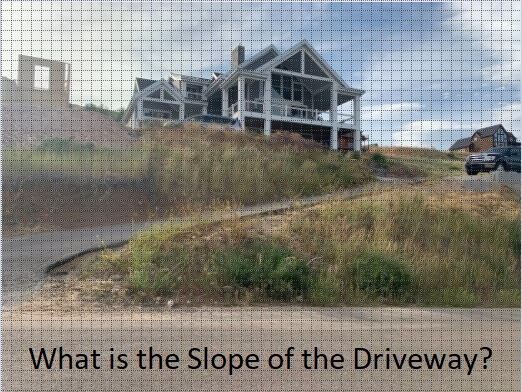# 1753 What Is the Slope of the Driveway?

Contents

### Today’s Puzzle:

With so much snow all around it is easy to think about summer. Last summer my family and I stayed at a magnificent Airbnb. There was more than enough room for my husband and me, our children and their spouses, and our grandchildren (32 people in total).

The owners of the Airbnb wrote that the best views come from homes with steep driveways. I will share some of those views later in the post. But just how steep was the driveway? I took a picture and added a grid so you can calculate the slope. Notice that the driveway zigzags. You can estimate where the front tire of the van parked at the top of the driveway hits the pavement to calculate the slope of the top part of the driveway.Here’s the top half of the driveway:

###The beautiful view from the top of the driveway:

###The next three pictures are gorgeous views from inside the house or from a balcony:

###The views definitely made the steep driveway worth it!

Since this is my 1753rd post, I’ll write a little about that number.

### Factors of 1753:

• 1753 is a prime number.
• Prime factorization: 1753 is prime.
• 1753 has no exponents greater than 1 in its prime factorization, so √1753 cannot be simplified.
• The exponent in the prime factorization is 1. Adding one to that exponent we get (1 + 1) = 2. Therefore 1753 has exactly 2 factors.
• The factors of 1753 are outlined with their factor pair partners in the graphic below.How do we know that 1753 is a prime number? If 1753 were not a prime number, then it would be divisible by at least one prime number less than or equal to √1753. Since 1753 cannot be divided evenly by 2, 3, 5, 7, 11, 13, 17, 19, 23, 29, 31, 37, or 41, we know that 1753 is a prime number.

### More About the Number 1753:

1753 is a powerful sum:
2¹º + 3⁶ = 1753.

Since both of those exponents are even, 1753 is also the sum of two squares:
32² + 27² = 1753.

1753 is the hypotenuse of a Pythagorean triple:
295-1728-1753, calculated from 32² – 27², 2(32)(27), 32² + 27².

Here’s another way we know that 1753 is a prime number: Since its last two digits divided by 4 leave a remainder of 1, and 32² + 27² = 1753 with 32 and 27 having no common prime factors, 1753 will be prime unless it is divisible by a prime number Pythagorean triple hypotenuse less than or equal to √1753. Since 1753 is not divisible by 5, 13, 17, 29, 37, or 41, we know that 1753 is a prime number.

## 7 thoughts on “1753 What Is the Slope of the Driveway?”

1.Charles Sanders

According to the fundamental theorem of arithmetic, any number can be factored into a product of distinct of distinct primes, including possible powers of those primes. Suppose that we look at the cardinality of of natural numbers, denoted aleph naught. Does this mean that aleph naught Is the only possible exception to the fundamental theorem?

•ivasallay

Thank you for writing this. I’ve sent an e-mail to you apologizing for my late response and explaining what prior knowledge I have or don’t have, unfortunately.

2.Charles Sanders

Aleph-naught was introduced by the German mathematician Georg Cantor, in his treatise “Contributions to the Foundations of Transfinite Numbers.”

3.Charles Sanders

Aleph-naught.was introduced by the German mathematician Georg Cantor, in his monumental treatise “Contributions to the Foundations of Transfinite Numbers.”

•ivasallay

Aleph-naught is an infinite set that is countable and aleph-one and so forth are uncountable sets?

4.rubber paving

Understanding the slope of your driveway is essential for both functionality and safety. It’s intriguing to see mathematics applied to everyday scenarios like driveways. This knowledge not only helps prevent water accumulation but also showcases the importance of basic math skills in practical situations.

5.paving West Hartford

The mathematical approach in ‘Find the Factors’ post adds an intriguing twist to understanding driveway slopes. The article’s uniqueness sparks reader engagement. Thanks for providing this valuable information.

This site uses Akismet to reduce spam. Learn how your comment data is processed.